## Introduction

We recognize that this package uses concepts that are not necessarily intuitive. As such, we offer a brief critique of proportionality analysis. Although the user may feel eager to start here, we strongly recommend first reading the companion vignette, “An Introduction to Proportionality”.

## Sample data

To facilitate discussion, we simulate count data for 5 features (e.g., genes) labeled “a”, “b”, “c”, “d”, and “e”, as measured across 100 subjects.

library(propr)
N <- 100
a <- seq(from = 5, to = 15, length.out = N)
b <- a * rnorm(N, mean = 1, sd = 0.1)
c <- rnorm(N, mean = 10)
d <- rnorm(N, mean = 10)
e <- rep(10, N)
X <- data.frame(a, b, c, d, e)


Let us assume that these data $$X$$ represent absolute abundance counts (i.e., not relative data). We can build a relative dataset, $$Y$$, by constraining and scaling $$X$$:

Y <- X / rowSums(X) * abs(rnorm(N))


## Spurious correlation

Next, we compare pairwise scatterplots for the absolute count data and the corresponding relative count data. We see quickly how these relative data suggest a spurious correlation: although genes “c” and “d” do not correlate with one another absolutely, their relative quantities do.

pairs(X) # absolute data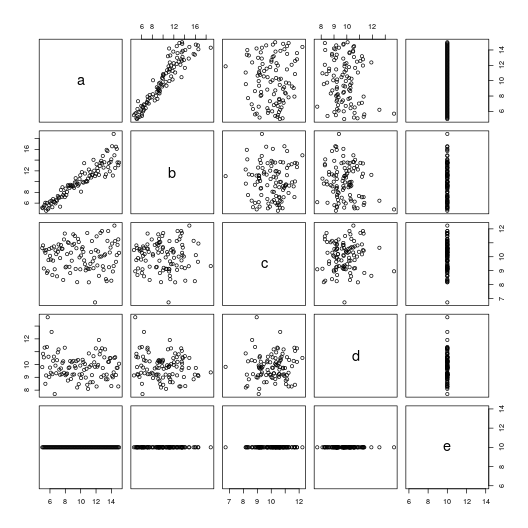pairs(Y) # relative data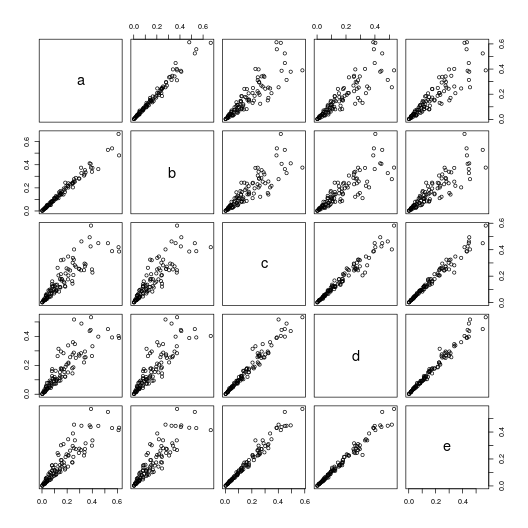Spurious correlation is evident by the correlation coefficients too.

suppressWarnings(cor(X)) # absolute correlation

##             a            b           c           d  e
## a  1.00000000  0.931788181 0.010043731 -0.13955429 NA
## b  0.93178818  1.000000000 0.003598548 -0.14819097 NA
## c  0.01004373  0.003598548 1.000000000  0.06939934 NA
## d -0.13955429 -0.148190975 0.069399336  1.00000000 NA
## e          NA           NA          NA          NA  1

cor(Y) # relative correlation

##           a         b         c         d         e
## a 1.0000000 0.9859307 0.8700492 0.8636699 0.8943947
## b 0.9859307 1.0000000 0.8667073 0.8613842 0.8862515
## c 0.8700492 0.8667073 1.0000000 0.9775640 0.9853551
## d 0.8636699 0.8613842 0.9775640 1.0000000 0.9879143
## e 0.8943947 0.8862515 0.9853551 0.9879143 1.0000000


## An in-depth look at VLR

In contrast, the variance of the log-ratios (VLR), defined as the variance of the logarithm of the ratio of two feature vectors, offers a measure of dependence that (a) does not change with respect to the nature of the data (i.e., absolute or relative), and (b) does not change with respect to the number of features included in the computation. As such, the VLR, constituting the numerator portion of the $$\phi$$ metric, and a portion of the $$\rho$$ metric as well, is considered sub-compositionally coherent. Yet, while VLR yields valid results for compositional data, it lacks a meaningful scale.

propr:::proprVLR(Y[, 1:4]) # relative VLR

##            a          b          c          d
## a 0.00000000 0.01097609 0.10948053 0.11693487
## b 0.01097609 0.00000000 0.12552455 0.13411702
## c 0.10948053 0.12552455 0.00000000 0.01890533
## d 0.11693487 0.13411702 0.01890533 0.00000000

propr:::proprVLR(X) # absolute VLR

##            a          b          c           d           e
## a 0.00000000 0.01097609 0.10948053 0.116934874 0.097960496
## b 0.01097609 0.00000000 0.12552455 0.134117023 0.114520860
## c 0.10948053 0.12552455 0.00000000 0.018905334 0.010851192
## d 0.11693487 0.13411702 0.01890533 0.000000000 0.009577957
## e 0.09796050 0.11452086 0.01085119 0.009577957 0.000000000


## An in-depth look at clr

In proportionality, we adjust the arbitrarily large VLR by the variance of its individual constituents. To do this, we need to place samples on a comparable scale. Log-ratio transformation, such as the centered log-ratio (clr) transformation, shifts the data onto a “standardized” scale that allows us to compare differences in the VLR-matrix.

In the next figures, we compare pairwise scatterplots for the clr-transformed absolute count data and the corresponding clr-transformed relative count data. While equivalent, we see a relationship between “c” and “d” that should not exist based on what we know from the non-transformed absolute count data. This demonstrates that, although the clr-transformation helps us compare values across samples, it does not rescue information lost by making absolute data relative.

pairs(propr:::proprCLR(Y[, 1:4])) # relative clr-transformation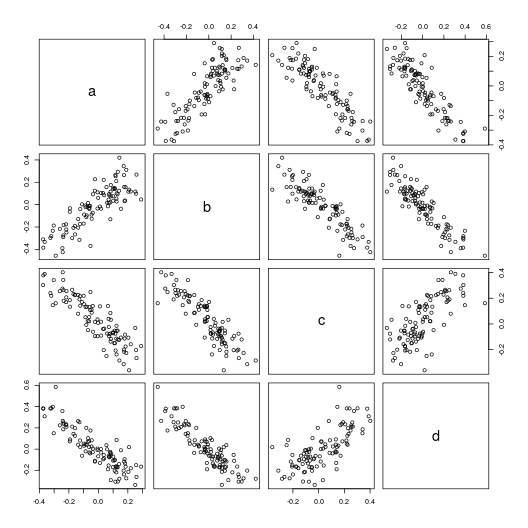pairs(propr:::proprCLR(X)) # absolute clr-transformation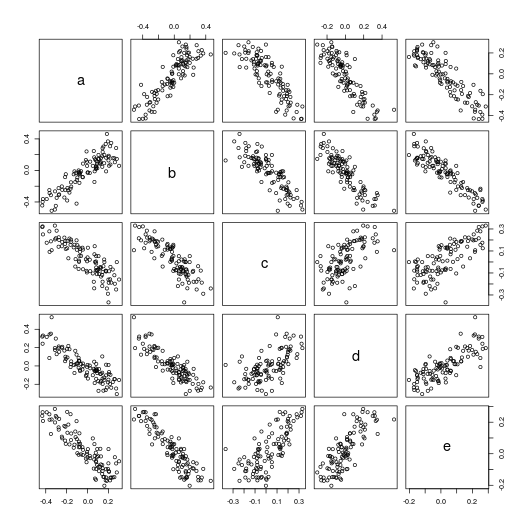Proportionality is a compromise between the advantages of VLR and the disadvantages of clr to establish a measure of dependence that is robust yet interpretable. As such, spurious proportionality is possible when the clr does not adequately approximate an ideal reference.

propr(Y[, 1:4])@matrix # relative proportionality with clr

##            a          b          c          d
## a  1.0000000  0.8244107 -0.8768141 -0.8756131
## b  0.8244107  1.0000000 -0.8836297 -0.8982919
## c -0.8768141 -0.8836297  1.0000000  0.7156007
## d -0.8756131 -0.8982919  0.7156007  1.0000000

propr(X)@matrix # absolute proportionality with clr

##            a          b          c          d          e
## a  1.0000000  0.8696275 -0.8211907 -0.8540631 -0.8227045
## b  0.8696275  1.0000000 -0.7913625 -0.8365445 -0.7977623
## c -0.8211907 -0.7913625  1.0000000  0.6137942  0.7261633
## d -0.8540631 -0.8365445  0.6137942  1.0000000  0.7750662
## e -0.8227045 -0.7977623  0.7261633  0.7750662  1.0000000


## An in-depth look at alr

The additive log-ratio (alr) adjusts each subject vector by the value of one its own components, chosen as a reference. If we select as a reference some feature $$D$$ with an a priori known fixed absolute count across all subjects, we can effectively “back-calculate” absolute data from relative data. When initially crafting the data $$X$$, we included “e” as this fixed value.

The following figures compare pairwise scatterplots for alr-transformed relative count data (i.e., $$\textrm{alr}(Y)$$ with “e” as the reference) and the corresponding absolute count data. We see here how the alr-transformation can eliminate the spurious correlation between “c” and “d”.

pairs(propr:::proprALR(Y, ivar = 5)) # relative alr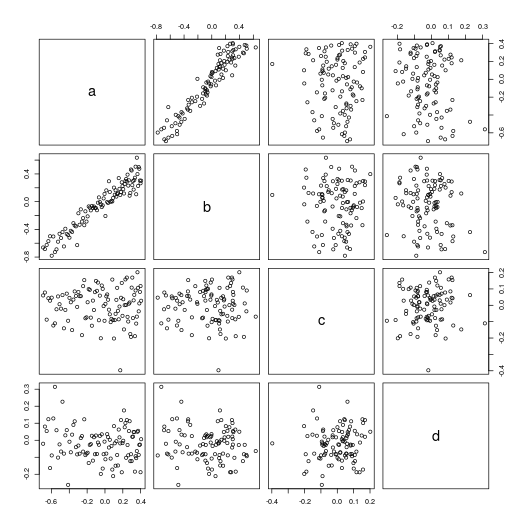pairs(X[, 1:4]) # absolute data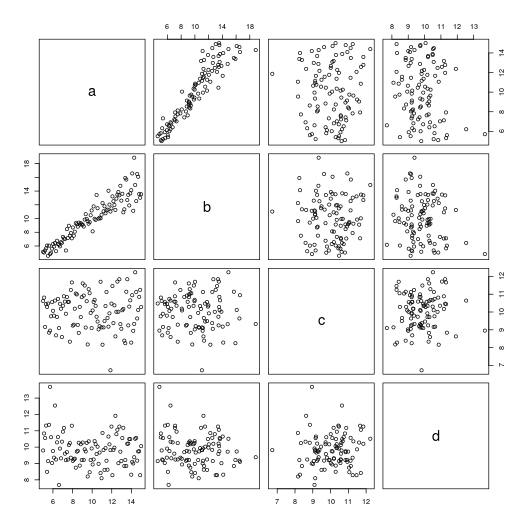Again, this gets reflected in the results of propr when we select “e” as the reference.

propr(Y, ivar = 5)@matrix # relative proportionality with alr

##              a            b            c           d e
## a  1.000000000  0.948343298 -0.006146749 -0.08737732 0
## b  0.948343298  1.000000000 -0.001216405 -0.08072766 0
## c -0.006146749 -0.001216405  1.000000000  0.07459021 0
## d -0.087377319 -0.080727657  0.074590210  1.00000000 0
## e  0.000000000  0.000000000  0.000000000  0.00000000 1